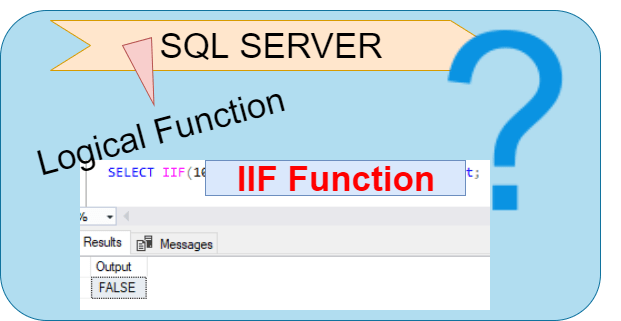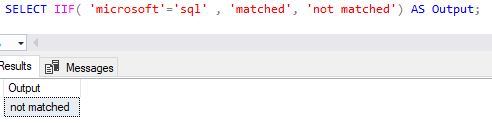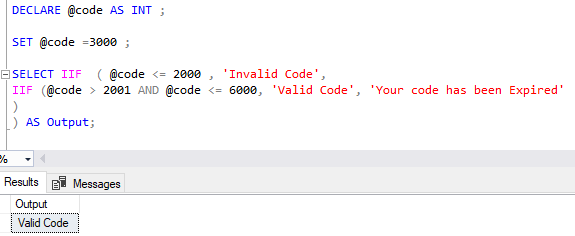Home » SQL Server IIF Function

# SQL Server IIF Function### SQL Server IIF function is the logical function that returns one of two parts, depending on the evaluation of an expression.

It evaluates the first argument and returns the second argument if the first argument is true; otherwise, it returns the second argument.

It also provides a easy way to write a Case Statement.

#### Syntax

`IIF (Boolean_Expression, True_Value, False_Value)`

boolean_expression

it is an expression to be evaluated.

true_value

It is the value to be returned, if the boolean_expression evaluates to true.

false_value

It is the value to be returned if the boolean_expression evaluates to false.

Lets look at an example using IIF Function to compare an Integer values.

Example 1:

Following statement uses IIF Function which evaluates the first argument 100 > 10 that is TRUE.  So, the second argument is returned as output, which is TRUE.

```SELECT IIF(100 > 10, 'TRUE', 'FALSE') AS Result;
```Example 2:
Following statement uses IIF Function which evaluates the first argument 10 > 100 that is FALSE. So, the Third argument is returned as output, which is FALSE.

```SELECT IIF(10 > 100, 'TRUE', 'FALSE') AS Output;

```Example 3:

Following statement uses IIF Function which evaluates the first argument that is ‘microsoft’ = ‘sql’  which is FALSE. So, the Third argument is returned as output, which is  ‘not matched

`SELECT IIF( 'microsoft'='sql' , 'matched', 'not matched') AS Output;`[Also Read logical function CHOOSE() ]

Example 4:
Following statement uses IIF Function which evaluates the first argument that is ‘sql’ = ‘sql’  which is TRUE. So, the second argument is returned as output, which is  ‘matched’.

`SELECT IIF( 'sql'='sql' , 'matched', 'not matched') AS Output;`#### Nested IIF Function

Following statement uses IIF function in nested way to evaluates the conditions and returns an output.

If input code is <= 2000 then it returns ‘invalid code’ , if code between 2001 and  6000 then it returns ‘valid code’ and if code greater than 6000 then it returns ‘your code has been expired’ .

```DECLARE @code AS INT ;
SET @code =1900 ;
SELECT IIF  ( @code <= 2000 , 'Invalid Code',
IIF (@code > 2001 AND @code <= 6000, 'Valid Code', 'Your code has been Expired'
)
) AS Output;```As we can see , it returns Invalid code , a code value is 1900 so the first argument check for condition @code <= 2000  that is true so, it returns second argument value for true  that is ‘invalid code’.

Lets try to pass code value 3000 in above statement.

```DECLARE @code AS INT ;

SET @code =3000 ;

SELECT IIF  ( @code <= 2000 , 'Invalid Code',
IIF (@code > 2001 AND @code <= 6000, 'Valid Code', 'Your code has been Expired'
)
) AS Output;

```As we can see, it returns valid code this time , a code value is 3000 so the first argument check for condition @code <= 3000  that is false so, it jumped on third argument and evaluates the condition @code > 2001 AND @code <= 6000 , code value 3000 satisfied this condition so it returns true value that is ‘valid code’.

CHOOSE()

DATEPART()

DATEDIFF()

GETDATE()

CURRENT_TIMESTAMP()

EOMONTH()